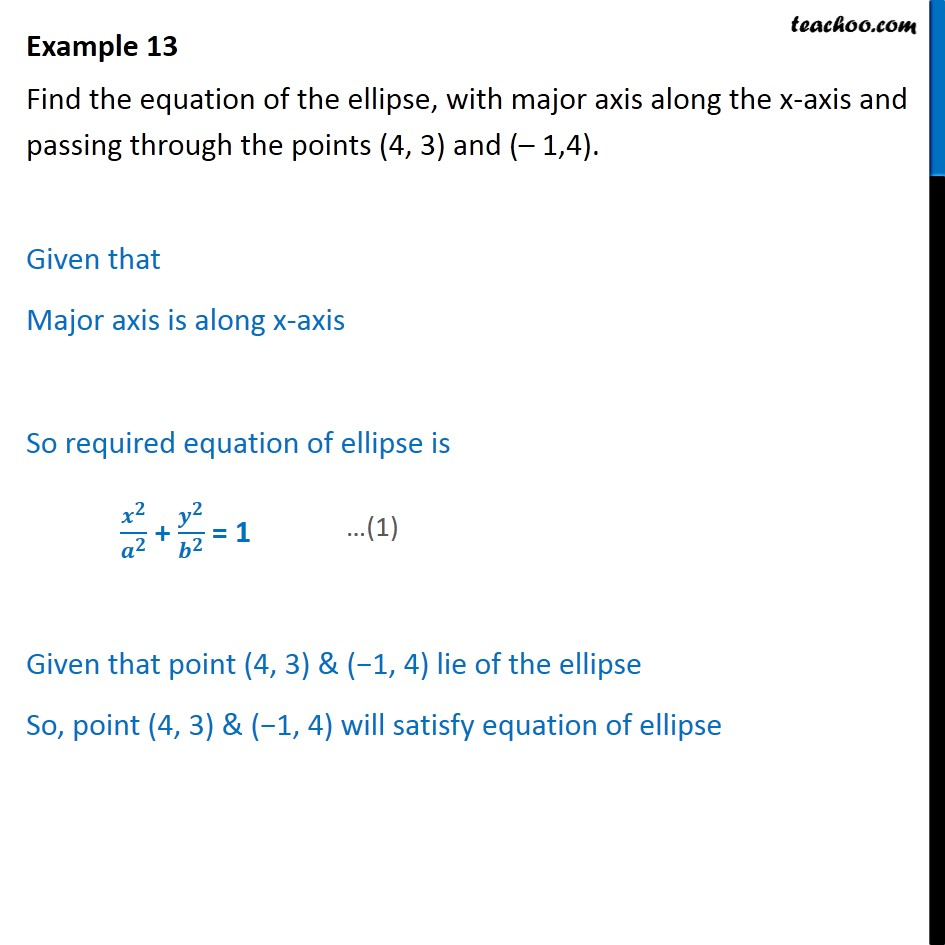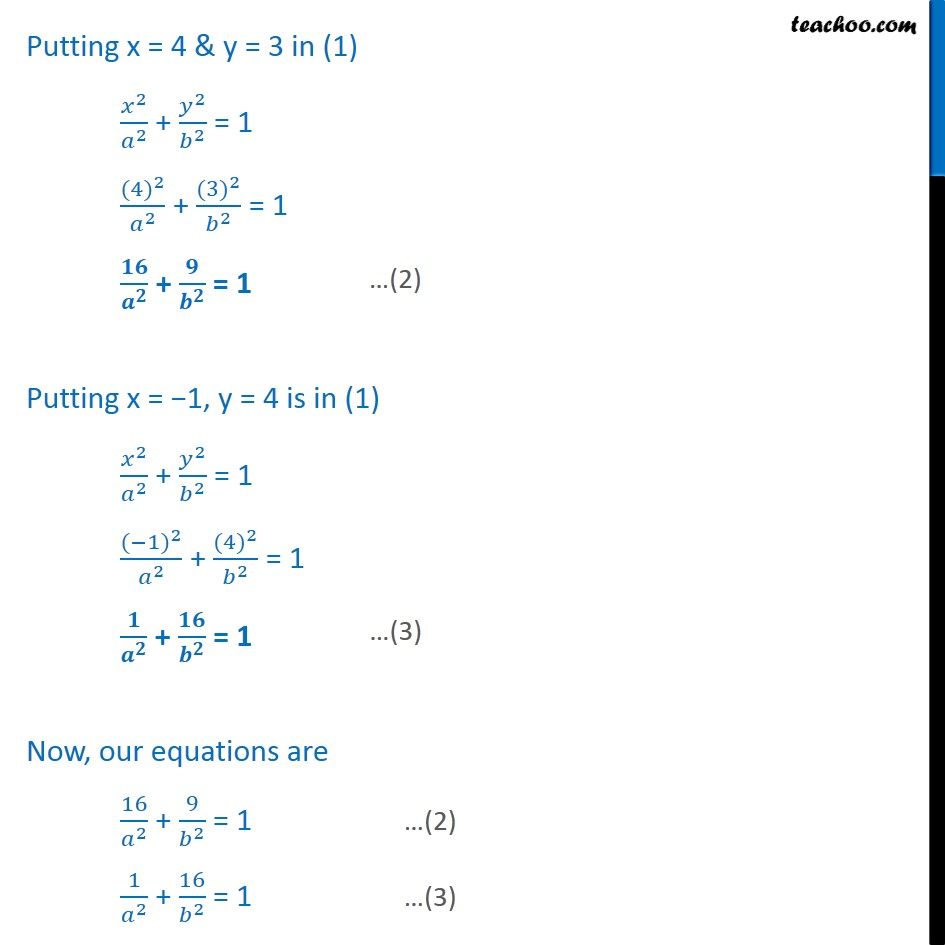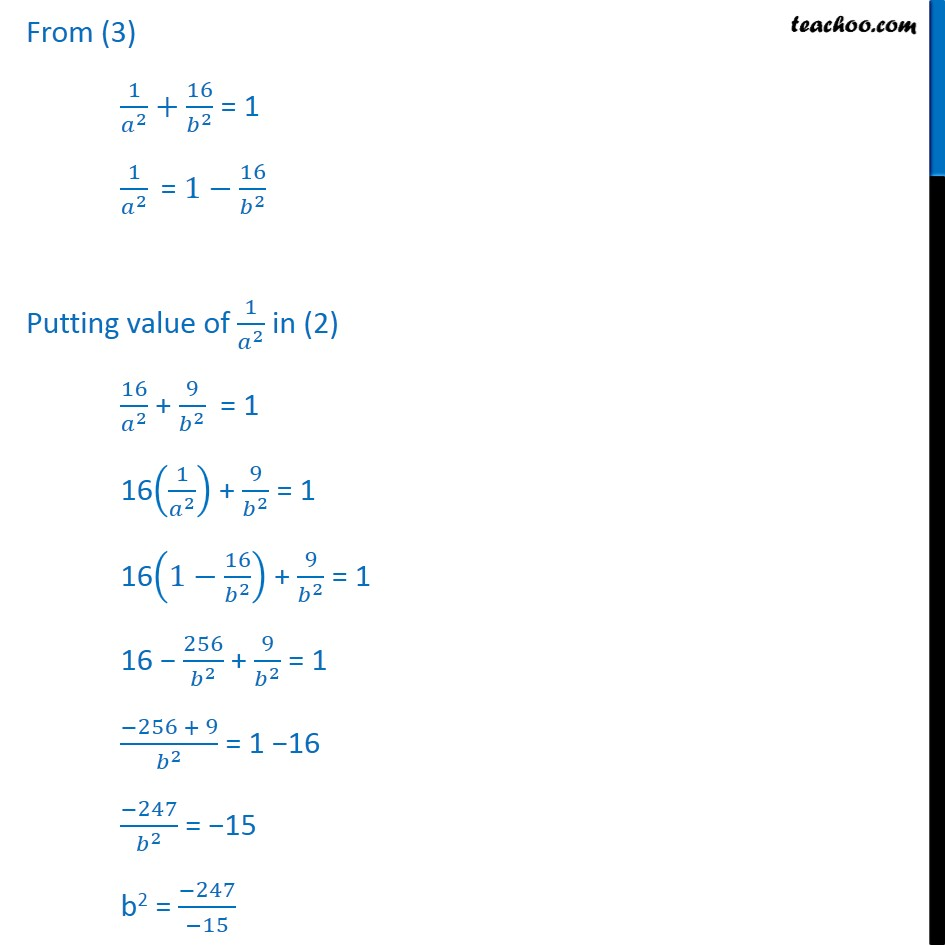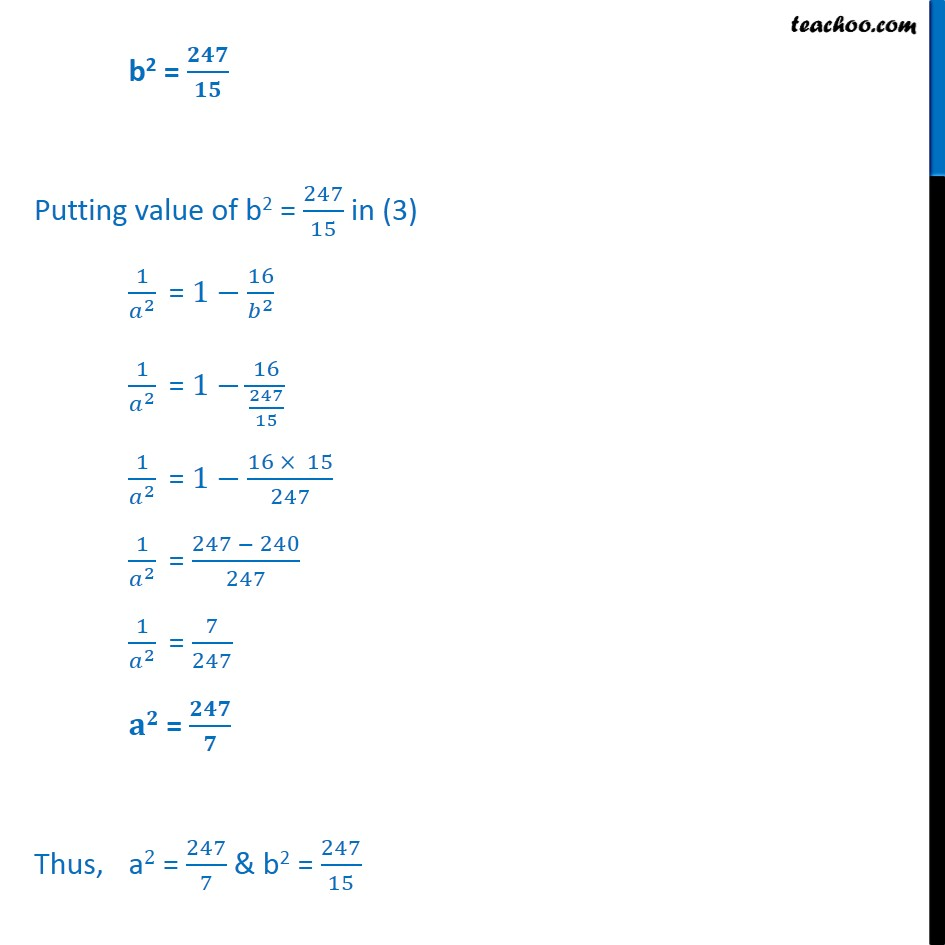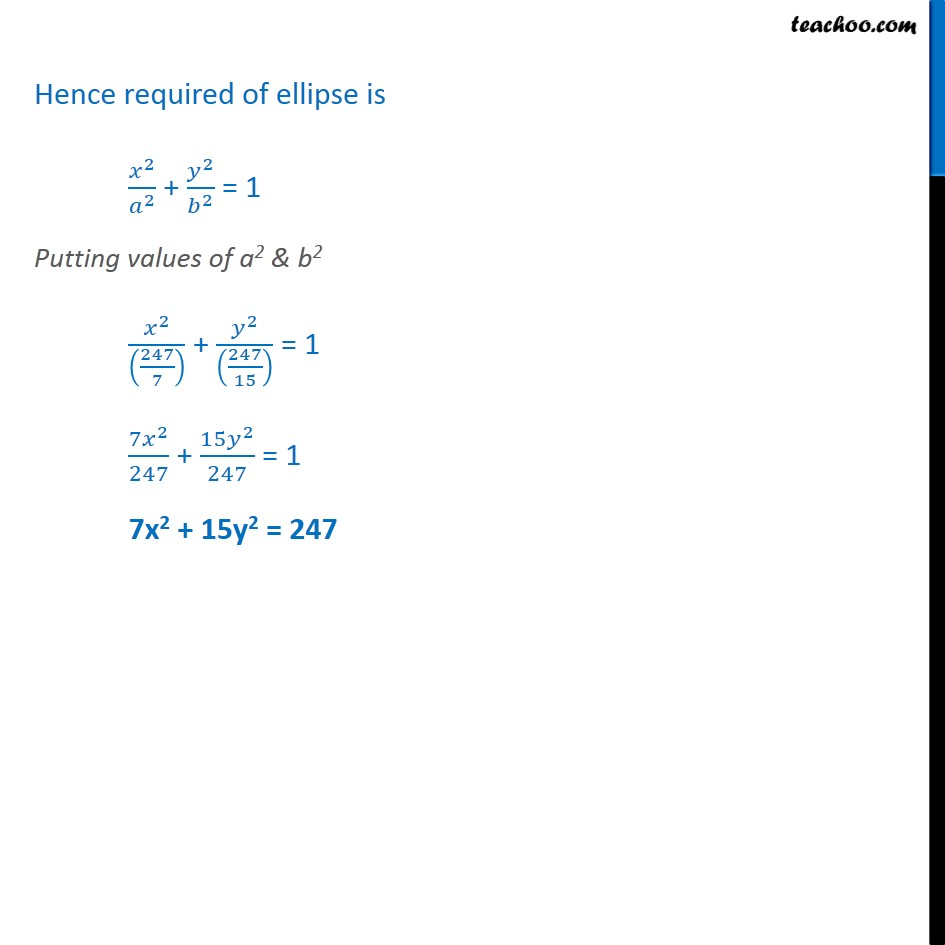1. Class 11
2. Important Question for exams Class 11
3. Chapter 11 Class 11 Conic Sections

Transcript

Example 13 Find the equation of the ellipse, with major axis along the x-axis and passing through the points (4, 3) and (– 1,4). Given that Major axis is along x-axis So required equation of ellipse is ﷐﷐𝒙﷮𝟐﷯﷮﷐𝒂﷮𝟐﷯﷯ + ﷐﷐𝒚﷮𝟐﷯﷮﷐𝒃﷮𝟐﷯﷯ = 1 Given that point (4, 3) & (−1, 4) lie of the ellipse So, point (4, 3) & (−1, 4) will satisfy equation of ellipse Putting x = 4 & y = 3 in (1) ﷐﷐𝑥﷮2﷯﷮﷐𝑎﷮2﷯﷯ + ﷐﷐𝑦﷮2﷯﷮﷐𝑏﷮2﷯﷯ = 1 ﷐﷐(4)﷮2﷯﷮﷐𝑎﷮2﷯﷯ + ﷐﷐(3)﷮2﷯﷮﷐𝑏﷮2﷯﷯ = 1 ﷐𝟏𝟔﷮﷐𝒂﷮𝟐﷯﷯ + ﷐𝟗﷮﷐𝒃﷮𝟐﷯﷯ = 1 Putting x = −1, y = 4 is in (1) ﷐﷐𝑥﷮2﷯﷮﷐𝑎﷮2﷯﷯ + ﷐﷐𝑦﷮2﷯﷮﷐𝑏﷮2﷯﷯ = 1 ﷐﷐(−1)﷮2﷯﷮﷐𝑎﷮2﷯﷯ + ﷐﷐(4)﷮2﷯﷮﷐𝑏﷮2﷯﷯ = 1 ﷐𝟏﷮﷐𝒂﷮𝟐﷯﷯ + ﷐𝟏𝟔﷮﷐𝒃﷮𝟐﷯﷯ = 1 Now, our equations are ﷐16﷮﷐𝑎﷮2﷯﷯ + ﷐9﷮﷐𝑏﷮2﷯﷯ = 1 …(2) ﷐1﷮﷐𝑎﷮2﷯﷯ + ﷐16﷮﷐𝑏﷮2﷯﷯ = 1 …(3) From (3) ﷐1﷮﷐𝑎﷮2﷯﷯+﷐16﷮﷐𝑏﷮2﷯﷯ = 1 ﷐1﷮﷐𝑎﷮2﷯﷯ = 1−﷐16﷮﷐𝑏﷮2﷯﷯ Putting value of ﷐1﷮﷐𝑎﷮2﷯﷯ in (2) ﷐16﷮﷐𝑎﷮2﷯﷯ + ﷐9﷮﷐𝑏﷮2﷯﷯ = 1 16﷐﷐1﷮﷐𝑎﷮2﷯﷯﷯ + ﷐9﷮﷐𝑏﷮2﷯﷯ = 1 16﷐1−﷐16﷮﷐𝑏﷮2﷯﷯﷯ + ﷐9﷮﷐𝑏﷮2﷯﷯ = 1 16 − ﷐256﷮﷐𝑏﷮2﷯﷯ + ﷐9﷮﷐𝑏﷮2﷯﷯ = 1 ﷐−256 + 9﷮﷐𝑏﷮2﷯﷯ = 1 −16 ﷐−247﷮﷐𝑏﷮2﷯﷯ = −15 b2 = ﷐−247﷮−15﷯ b2 = ﷐𝟐𝟒𝟕﷮𝟏𝟓﷯ Putting value of b2 = ﷐247﷮15﷯ in (3) ﷐1﷮﷐𝑎﷮2﷯﷯ = 1−﷐16﷮﷐𝑏﷮2﷯﷯ ﷐1﷮﷐𝑎﷮2﷯﷯ = 1−﷐16﷮﷐247﷮15﷯﷯ ﷐1﷮﷐𝑎﷮2﷯﷯ = 1−﷐16 × 15﷮247﷯ ﷐1﷮﷐𝑎﷮2﷯﷯ = ﷐247 − 240﷮247﷯ ﷐1﷮﷐𝑎﷮2﷯﷯ = ﷐7﷮247﷯ 𝐚𝟐 = ﷐𝟐𝟒𝟕﷮𝟕﷯ Thus, a2 = ﷐247﷮7﷯ & b2 = ﷐247﷮15﷯ Hence required of ellipse is ﷐﷐𝑥﷮2﷯﷮﷐𝑎﷮2﷯﷯ + ﷐﷐𝑦﷮2﷯﷮﷐𝑏﷮2﷯﷯ = 1 Putting values of a2 & b2 ﷐﷐𝑥﷮2﷯﷮﷐﷐247﷮7﷯﷯﷯ + ﷐﷐𝑦﷮2﷯﷮﷐﷐247﷮15﷯﷯﷯ = 1 ﷐7﷐𝑥﷮2﷯﷮247﷯ + ﷐15﷐𝑦﷮2﷯﷮247﷯ = 1 7x2 + 15y2 = 247

Chapter 11 Class 11 Conic Sections

Class 11
Important Question for exams Class 11